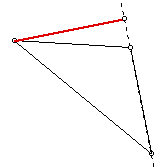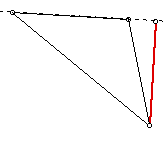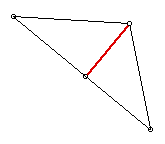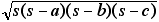# Area Formulas

Use a geometry software to construct a triangle and measure its area.

Measure the length of one side (a base) and the altitude (a height) that is drawn to that side. Use these measurements to determine a formula for the area of the triangle.Will the formula give the same result if you use a different side of the triangle for the base and the altitude that corresponds to that base? Explain.Extension
The semiperimeter is equal to one half of the perimeter of a triangle. About 2000 years ago, Heron of Alexandria discovered that the area (A) of a triangle is related to the semiperimeter (s), and each of the three sides (a, b, and c) by the following formula:Use a geometry software to verify that this formula is correct. Here’s a proof of Heron’s formula demonstrated by James W. Wilson.